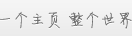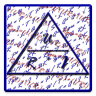# 手机助手

## 本类热门应用## EA free Voltage drop

6.0 0条评价 下载：3次 2.69M
应用介绍
Electrician App - is a good companion for an electrician or electronics engineer in training, on the job or for hobby electricians and handymen.This is a Free-Version.Functions of the Free-Version:Calculating the voltage drop on the line.Electrician App contains the most important formulas in the field of electrical engineering. Easy to use, available in 3 languages​​: English, German and Russian.Features of Electrician App:Unloaded voltage divider​​.Loaded voltage divider​​.Flow divider.Calculation of up to twenty resistors (parallel).Calculation of up to twenty resistors (in series).Calculation of resistors (mixed).Calculation of current, charge and time.Calculation of work, time and power.Calculation of line resistance.Calculation of current, voltage, power and resistance.Calculating the voltage drop on the line.Calculating the maximum length of the conductor.Calculation of the conductor cross-section.Calculation of real, reactive, apparent power at AC.Calculation of real, reactive, apparent power for three-phase.Calculation of primary voltage, secondary voltage, primary windings, secondary windings of a transformer.Calculating the cross section and diameter.Calculation of the current density.Calculation of cos φ.Calculation of sin φ.For the accuracy of the calculation, we assume no liability.Forget complicated formulas and calculation methods, specify a value-known and Electrician App returns the result. Several updates and new formulas more languages ​​are planned in the near future.Electrician App is optimized and improved by us and as for we depend on your help.Please add comments, write your opinions, suggestions or report any errors.Thanks in advance!

【基本信息】
 作者：fellex 更新时间：2015-01-01 版本：1.4 系统：Android 1.6以上 语言：其他

展开该应用暂时还没有评论，沙发空缺中。
抢先评论 >>

## 应用评分：6.0分

份评分

这款应用褒贬不一
自己试试看吧

请先下载此应用，才可对其评分。

我来评分：

- 有内置广告

- 免费

- 访问网络
- 获取网络状态